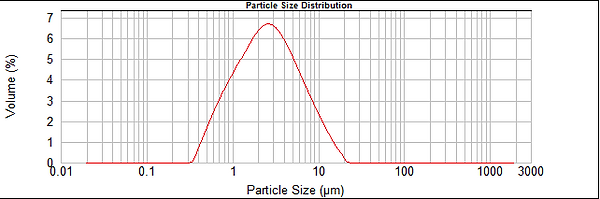top of page## Nutrients, Herbs, Natural Products

We have gained a lot of experience in micronization of food additives. Being soft and delicate, other milling or micronization techniques may require pre-cooling of the feed or cryogenic milling. SuperFine’s technology can handle most products in room temperature. Examples:

Egg Shell –

d(10)= 0.9   d(50)=3.0 d(90)=6.9Vitamin A –

d(10)=3.5  d(50)=13  d(90)= 26Microcristaline Cellulose –

d(10)=1.55 d(50)=4.2 d(90)=9.08Seaweed Red  –

d(10)=1.24 d(50)=4.28 d(90)=7.93Soya Protein –

d(10)=4.2 d(50)=11.8 d(90)=23.2Lactose  –

d(10)=0.8  d(50)=2.54 d(90)=7.96Three Zink Citrate –

d(10)=1.17   d(50)=2.85  d(90)=7.23Parsley  –

d(10)=1.76    d(50)=5.12    d(90)=11.2Phytrol  –

d(10)=1.42  d(50)=3.56  d(90)=7.08Phytosterol  –

d(10)=1.04  d(50)=2.85  d(90)=6.37bottom of page